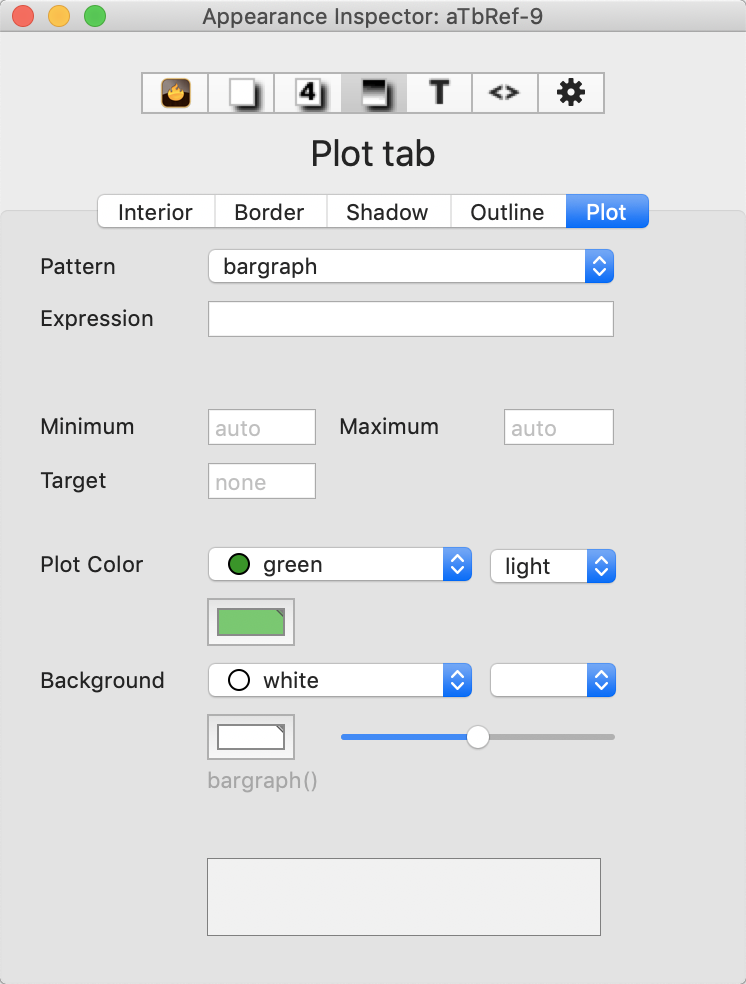# Plot tabThis tab gives controls for setting calculated patterns, such as for container plots.

Pattern. The type of container plot expression. A pop-up list shows the options available. Static patterns are listed but should be ignored as they are not pertinent here.

Expression. The container plot expression (code), i.e. the input argument used by the plot type. The input box and label are hidden if the selected pattern type does not support expression.

X axis. Only visible if xyplot() is the selected plot type. Second input argument for the xyplot() expression.

Minimum. Optional maximum value for the Y axis. Only shown if a dynamic plot type is selected.

Maximum. Optional maximum value for the Y axis. Only shown if a dynamic plot type is selected.

Target. Optional value at which dotted line is drawn in \$PlotColor. Only shown if the plot type is bar() or vbar(). Shown for all plot types except pie().

Plot Color. Tinderbox colour controls to set the colour of the plotted data in a container plot (\$PlotColor).

Background. Tinderbox colour controls to set the colour of the container plot background for this note's Map (\$PlotBackgroundColor). The slider is used to set the opacity of the plot's background; if transparent, the child map shows through (\$PlotBackgroundOpacity).

Defined plot pattern. Grey text caption with the current contents of \$Pattern, as defined by the settings above.

Preview window. A preview image of the above settings as a plot.